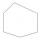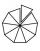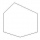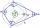# Adding + polygon - math problems

#### Number of problems found: 5

• Six-sided polygonIn a six-sided polygon. The first two angles are equal, the third angle is twice (the equal angles), two other angles are trice the equal angle, while the last angle is a right angle. Find the value of each angle.
• 9-gonSum of interiol angles of 9-gon is:
• Angles of a hexagonFind the interior angles of a hexagon if the sizes of the angles form an arithmetic sequence and the smallest angle is 70°.What size have angles in quadrilateral (4-gon) if they are in a ratio of 8: 9: 10: 13?On each wall of a regular octahedron is written one of the numbers 1, 2, 3, 4, 5, 6, 7 and 8, wherein on different sides are different numbers. For each wall John make the sum of the numbers written of three adjacent walls. Thus got eight sums, which also